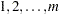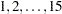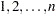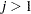Specifying Factor Structures

By appropriately combining features of the PLAN procedure, you can construct an extensive set of designs. The basic tools are the factor-selections, which are used in the FACTORS and TREATMENTS statements. Table 67.1 summarizes how the procedure interprets various factor-selections (assuming that the ORDERED option is not specified in the PROC PLAN statement).

Table 67.1 Factor-Selection Interpretation

Form of

Request

Interpretation

Example

Results

name=produce a random permutation of the integerst=15

lists a random ordering of the numbersname=cyclic

cyclically permute the integerst=5 cyclic

selects the integers 1 to 5. On the next iteration, selects 2,3,4,5,1; then 3,4,5,1,2; and so on.

name=ofchoose a random sample ofintegers (without replacement) from the set of integerst=5 of 15

lists a random selection of 5 numbers from 1 to 15. First, the procedure selects 5 numbers and then arranges them in random order.

name=ofordered

has the same effect as name=ordered

t=5 of 15
ordered

lists the integers 1 to 5 in increasing order (same as t=5 ordered)

name=ofcyclic

permuteof theintegers

t=5 of 30
cyclic

selects the integers 1 to 5. On the next iteration, selects 2,3,4,5,6; then 3,4,5,6,7; and so on. The 30th iteration produces 30,1,2,3,4; the 31st iteration produces 1,2,3,4,5; and so on.

name=perm

produce a list of all permutations ofintegers

t=5 perm

lists the integers 1,2,3,4,5 on the first iteration; on the second lists 1,2,3,5,4; on the 119th iteration lists 5,4,3,1,2; and on the last (120th) lists 5,4,3,2,1.

name=ofcomb

choose combinations ofintegers fromintegers

t=3 of 5
comb

lists all combinations of 5 choose 3 integers. The first iteration is 1,2,3; the second is 1,2,4; the third is 1,2,5; and so on until the last iteration 3,4,5.

name=ofcyclic
(initial-block)

permuteof theintegers, starting with the values specified in the initial-block

t=4 of 30
cyclic
(2 10 15 18)

selects the integers 2,10,15,18. On the next iteration, selects 3,11,16,19; then 4,12,17,20; and so on. The thirteenth iteration is 14,22,27,30; the fourteenth iteration is 15,23,28,1; and so on.

name=ofcyclic
(initial-block)
increment

permuteof theintegers. Start with the values specified in the initial-block, then add the increment to each value.

t=4 of 30
cyclic
(2 10 15 18)
2

selects the integers 2,10,15,18. On the next iteration, selects 4,12,17,20; then 6,14,19,22; and so on. The wrap occurs at the eighth iteration. The eighth iteration is 16,24,29,2; and so on.

In Table 67.1, in order for more than one iteration to appear in the plan, another name=j factor selection (with) must precede the example factor selection. For example, the following statements produce six of the iterations described in the last entry of Table 67.1.

```proc plan;
factors c=6 ordered t=4 of 30 cyclic (2 10 15 18) 2;
run;
```

The following statements create a randomized complete block design and output the design to a data set.

```proc plan ordered;
factors blocks=3 cell=5;
treatments t=5 random;
output out=rcdb;
run;
```